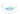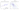## The Cost Function

We can measure the accuracy of our hypothesis function by using a cost function.

The cost function $J(\theta_0, \theta_1)$ is defined as:

$J(\theta_0, \theta_1) = \dfrac {1}{2m} \displaystyle \sum _{i=1}^m \left ( \hat{y}^{(i)}- y^{(i)} \right)^2 = \dfrac {1}{2m} \displaystyle \sum _{i=1}^m \left (h_\theta (x^{(i)}) - y^{(i)} \right)^2$

The cost function is actually $\frac{1}{2}\bar{x}$ where $\bar{x}$ is "Mean squared error (MSE)" or "Squared error function". The mean is halved as a convenience for the computation of the gradient descent, as the derivative term of the square function will cancel out the $\frac{1}{2}$ term.

If we try to think of it in visual terms, our training data set is scattered on the x-y plane. We are trying to make straight line (defined by $h_\theta(x)$ which passes through this scattered set of data. Our objective is to get the best possible line. The best possible line will be such so that the cost function $J(\theta_0, \theta_1)$ is minimized with respect to $\theta_0$ and $\theta_1$.

The cost function is sometimes also called optimization function or objective function.A contour plot is a graph that contains many contour lines. A contour line of a two variable function has a constant value at all points of the same line. Taking any color and going along the 'circle', one would expect to get the same value of the cost function.#### Notations

• $m$ : Number of training examples
• $x$ : Input variable
• $y$ : Output variable / Target variable
• $(x, y)$: Training examples
• $(x^{(i)}, y^{(i)})$: $i$th training example
• $h$: Hypothesis function
• $\theta_i$'s: parameters of the model